###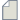Author Topic: error  (Read 201 times)

0 Members and 1 Guest are viewing this topic.

####A_Shimizu

• New ATK user
•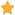• Posts: 4
• Country: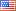• Reputation: 0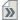##### error
« on: July 15, 2021, 19:46 »
Hello
I have calculated Co3O4 for Band structure and projected DOS. I am seeing this message and stopped calculations.

bulk_configuration = OptimizeGeometry(
File "zipdir\NL\Dynamics\Optimization\OptimizeGeometry.py", line 507, in OptimizeGeometry
Traceback (most recent call last):
Traceback (most recent call last):
89, in <module>
Traceback (most recent call last):
File "Co3 O4 +U 1.25eV.py", line 389, in <module>
File "Co3 O4 +U 1.25eV.py", line 3Traceback (most recent call last):
89, in <module>
Traceback (most recent call last):
89, in <module>
Traceback (most recent call last):
File "Co3 O4 +U 1.25eV.py", line 389, in <module>
File "Co3 O4 +U 1.25eV.py", line 389, in <module>
Traceback (most recent call last):
Traceback (most recent call last):
89, in <module>
File "Co3 O4 +U 1.25eV.py", line 389, in <module>
File "Co3 O4 +U 1.25eV.py", line 389, in <module>
Traceback (most recent call last):
File "Co3 O4 +U 1.25eV.py", line 389, in <module>
File "Co3 O4 +U 1.25eV.py", line 389, in <module>
File "Co3 O4 +U 1.25eV.py", line 389, in <module>
File "zipdir\NL\Dynamics\Optimization\OptimizeGeometry.py", line 507, in OptimizeGeometry
Traceback (most recent call last):
File "Co3 O4 +U 1.25eV.py", line 389, in <module>
bulk_configuration = OptimizeGeometry(
File "zipdir\NL\Dynamics\Optimization\OptimizeGeometry.py", line 507, in OptimizeGeometry
bulk_configuration = OptimizeGeometry(
File "zipdir\NL\Dynamics\Optimization\OptimizeGeometry.py", line 507, in OptimizeGeometry
bulk_configuration = OptimizeGeometry(
bulk_configuration = OptimizeGeometry(
File "zipdir\NL\Dynamics\Optimization\OptimizeGeometry.py", line 507, in OptimizeGeometry
bulk_configuration = OptimizeGeometry(
mizeGeometry.py", line 507, in OptimizeGeometry
File "zipdir\NL\Dynamics\Optimization\OptimizeGeometry.py", line 507, in OptimizeGeometry
File "zipdir\NL\Dynamics\Optimization\OptimizeGeometry.py", line 507, in OptimizeGeometry
bulk_configuration = OptimizeGeometry(
bulk_configuration = OptimizeGeometry(
mizeGeometry.py", line 507, in OptimizeGeometry
File "zipdir\NL\Dynamics\Optimization\OptimizeGeometry.py", line 507, in OptimizeGeometry
File "zipdir\NL\Dynamics\Optimization\OptimizeGeometry.py", line 507, in OptimizeGeometry
bulk_configuration = OptimizeGeometry(
bulk_configuration = OptimizeGeometry(
File "zipdir\NL\Dynamics\Optimization\OptimizeGeometry.py", line 507, in OptimizeGeometry
File "zipdir\NL\Dynamics\Optimization\OptimizeGeometry.py", line 507, in OptimizeGeometry
bulk_configuration = OptimizeGeometry(
File "zipdir\NL\Dynamics\Optimization\OptimizeGeometry.py", line 507, in OptimizeGeometry
File "zipdir\NL\Dynamics\Optimization\OptimizeGeometry.py", line 1137, in runRelaxation
File "zipdir\NL\Dynamics\Optimization\OptimizeGeometry.py", line 716, in atomicForces
File "zipdir\NL\Dynamics\Optimization\OptimizeGeometry.py", line 1137, in runRelaxation
File "zipdir\NL\Dynamics\Optimization\OptimizeGeometry.py", line 1137, in runRelaxation
File "zipdir\NL\Dynamics\Optimization\OptimizeGeometry.py", line 1137, in runRelaxation
File "zipdir\NL\Dynamics\Optimization\OptimizeGeometry.py", line 1137, in runRelaxation
File "zipdir\NL\Dynamics\Optimization\OptimizeGeometry.py", line 1137, in runRelaxation
File "zipdir\NL\Dynamics\Optimization\OptimizeGeometry.py", line 1137, in runRelaxation
File "zipdir\NL\Dynamics\Optimization\OptimizeGeometry.py", line 1137, in runRelaxation
File "zipdir\NL\Dynamics\Optimization\OptimizeGeometry.py", line 1137, in runRelaxation
File "zipdir\NL\Dynamics\Optimization\OptimizeGeometry.py", line 1137, in runRelaxation
File "zipdir\NL\Dynamics\Optimization\OptimizeGeometry.py", line 1137, in runRelaxation
File "zipdir\NL\Dynamics\Optimization\OptimizeGeometry.py", line 1137, in runRelaxation
File "zipdir\NL\Dynamics\Optimization\OptimizeGeometry.py", line 1137, in runRelaxation
File "zipdir\NL\Dynamics\Optimization\OptimizeGeometry.py", line 637, in _update
File "zipdir\NL\Dynamics\Optimization\OptimizeGeometry.py", line 716, in atomicForces
File "zipdir\NL\Dynamics\Optimization\OptimizeGeometry.py", line 716, in atomicForces
File "zipdir\NL\Dynamics\Optimization\OptimizeGeometry.py", line 716, in atomicForces
File "zipdir\NL\Dynamics\Optimization\OptimizeGeometry.py", line 716, in atomicForces
File "zipdir\NL\Dynamics\Optimization\OptimizeGeometry.py", line 716, in atomicForces
File "zipdir\NL\Dynamics\Optimization\OptimizeGeometry.py", line 716, in atomicForces
File "zipdir\NL\Dynamics\Optimization\OptimizeGeometry.py", line 716, in atomicForces
File "zipdir\NL\Dynamics\Optimization\OptimizeGeometry.py", line 716, in atomicForces
File "zipdir\NL\Dynamics\Optimization\OptimizeGeometry.py", line 716, in atomicForces
File "zipdir\NL\Dynamics\Optimization\OptimizeGeometry.py", line 716, in atomicForces
File "zipdir\NL\Dynamics\MolecularDynamics\DynamicsEnergyForcesStress.py", line 30, in wrapped_analysis
File "zipdir\NL\Dynamics\Optimization\OptimizeGeometry.py", line 716, in atomicForces
File "zipdir\NL\Dynamics\Optimization\OptimizeGeometry.py", line 716, in atomicForces
File "zipdir\NL\Dynamics\Optimization\OptimizeGeometry.py", line 716, in atomicForces
File "zipdir\NL\Dynamics\Optimization\OptimizeGeometry.py", line 716, in atomicForces
File "zipdir\NL\Dynamics\Optimization\OptimizeGeometry.py", line 637, in _update
File "zipdir\NL\Calculators\DensityFunctionalTheory\LCAOCalculator\Analysis\FastEnergyForcesStress.py", line 34, in energyFunction
File "zipdir\NL\Dynamics\Optimization\OptimizeGeometry.py", line 637, in _update
File "zipdir\NL\Dynamics\Optimization\OptimizeGeometry.py", line 637, in _update
File "zipdir\NL\Dynamics\Optimization\OptimizeGeometry.py", line 637, in _update
File "zipdir\NL\Dynamics\Optimization\OptimizeGeometry.py", line 637, in _update
File "zipdir\NL\Dynamics\Optimization\OptimizeGeometry.py", line 637, in _update
File "zipdir\NL\Dynamics\Optimization\OptimizeGeometry.py", line 637, in _update
File "zipdir\NL\Dynamics\Optimization\OptimizeGeometry.py", line 637, in _update
File "zipdir\NL\Dynamics\Optimization\OptimizeGeometry.py", line 637, in _update
File "zipdir\NL\Dynamics\Optimization\OptimizeGeometry.py", line 637, in _update
File "zipdir\NL\Dynamics\Optimization\OptimizeGeometry.py", line 637, in _update
File "zipdir\NL\Dynamics\MolecularDynamics\DynamicsEnergyForcesStress.py", line 30, in wrapped_analysis
File "zipdir\NL\Dynamics\MolecularDynamics\DynamicsEnergyForcesStress.py", line 30, in wrapped_analysis
File "zipdir\NL\Calculators\DensityFunctionalTheory\LCAOCalculator\Analysis\FastEnergyForcesStress.py", line 71, in _checkAndUpdate
File "zipdir\NL\Dynamics\MolecularDynamics\DynamicsEnergyForcesStress.py", line 30, in wrapped_analysis
File "zipdir\NL\Dynamics\MolecularDynamics\DynamicsEnergyForcesStress.py", line 30, in wrapped_analysis
File "zipdir\NL\Dynamics\MolecularDynamics\DynamicsEnergyForcesStress.py", line 30, in wrapped_analysis
File "zipdir\NL\Dynamics\MolecularDynamics\DynamicsEnergyForcesStress.py", line 30, in wrapped_analysis
File "zipdir\NL\Dynamics\MolecularDynamics\DynamicsEnergyForcesStress.py", line 30, in wrapped_analysis
File "zipdir\NL\Dynamics\MolecularDynamics\DynamicsEnergyForcesStress.py", line 30, in wrapped_analysis
File "zipdir\NL\Dynamics\MolecularDynamics\DynamicsEnergyForcesStress.py", line 30, in wrapped_analysis
File "zipdir\NL\Dynamics\MolecularDynamics\DynamicsEnergyForcesStress.py", line 30, in wrapped_analysis
File "zipdir\NL\Dynamics\MolecularDynamics\DynamicsEnergyForcesStress.py", line 30, in wrapped_analysis
File "zipdir\NL\Dynamics\MolecularDynamics\DynamicsEnergyForcesStress.py", line 30, in wrapped_analysis
File "zipdir\NL\Dynamics\MolecularDynamics\DynamicsEnergyForcesStress.py", line 30, in wrapped_analysis
File "zipdir\NL\Calculators\DensityFunctionalTheory\LCAOCalculator\Analysis\FastEnergyForcesStress.py", line 103, in _update
File "zipdir\NL\Calculators\DensityFunctionalTheory\LCAOCalculator\Analysis\FastEnergyForcesStress.py", line 34, in energyFunction
File "zipdir\NL\Calculators\DensityFunctionalTheory\LCAOCalculator\Analysis\FastEnergyForcesStress.py", line 34, in energyFunction
File "zipdir\NL\Calculators\DensityFunctionalTheory\LCAOCalculator\Analysis\FastEnergyForcesStress.py", line 34, in energyFunction
File "zipdir\NL\Calculators\DensityFunctionalTheory\LCAOCalculator\Analysis\FastEnergyForcesStress.py", line 34, in energyFunction
File "zipdir\NL\Calculators\DensityFunctionalTheory\LCAOCalculator\Analysis\FastEnergyForcesStress.py", line 34, in energyFunction
File "zipdir\NL\Calculators\DensityFunctionalTheory\LCAOCalculator\Analysis\FastEnergyForcesStress.py", line 34, in energyFunction
zipdir\NL\Calculators\DensityFunctionalTheory\LCAOCalculator\Analysis\FastEnergyForcesStress.py", line 34, in energyFunction
File "zipdir\NL\Calculators\DensityFunctionalTheory\LCAOCalculator\Analysis\FastEnergyForcesStress.py", line 34, in energyFunction
File "zipdir\NL\Calculators\DensityFunctionalTheory\LCAOCalculator\Analysis\FastEnergyForcesStress.py", line 34, in energyFunction
File "zipdir\NL\Calculators\DensityFunctionalTheory\LCAOCalculator\Analysis\FastEnergyForcesStress.py", line 34, in energyFunction
File "zipdir\NL\Calculators\DensityFunctionalTheory\LCAOCalculator\Analysis\FastEnergyForcesStress.py", line 34, in energyFunction
File "zipdir\NL\Calculators\DensityFunctionalTheory\LCAOCalculator\Analysis\FastEnergyForcesStress.py", line 34, in energyFunction
File "zipdir\NL\Calculators\DensityFunctionalTheory\LCAOCalculator\Analysis\FastEnergyForcesStress.py", line 34, in energyFunction
File "zipdir\NL\Calculators\DensityFunctionalTheory\LCAOCalculator\Analysis\FastEnergyForcesStress.py", line 71, in _checkAndUpdate
File "zipdir\NL\Calculators\DensityFunctionalTheory\LCAOCalculator\Analysis\FastEnergyForcesStress.py", line 71, in _checkAndUpdate
File "zipdir\NL\Calculators\DensityFunctionalTheory\LCAOCalculator\Analysis\FastEnergyForcesStress.py", line 71, in _checkAndUpdate
File "zipdir\NL\Calculators\DensityFunctionalTheory\LCAOCalculator\Analysis\FastEnergyForcesStress.py", line 71, in _checkAndUpdate
File "zipdir\NL\Calculators\CounterpoiseCorrector\CounterpoiseCorrector.py", line 830, in _checkConfiguration
File "zipdir\NL\Calculators\DensityFunctionalTheory\LCAOCalculator\Analysis\FastEnergyForcesStress.py", line 71, in _checkAndUpdate
File "zipdir\NL\Calculators\DensityFunctionalTheory\LCAOCalculator\Analysis\FastEnergyForcesStress.py", line 71, in _checkAndUpdate
File "zipdir\NL\Calculators\DensityFunctionalTheory\LCAOCalculator\Analysis\FastEnergyForcesStress.py", line 71, in _checkAndUpdate
File "zipdir\NL\Calculators\DensityFunctionalTheory\LCAOCalculator\Analysis\FastEnergyForcesStress.py", line 71, in _checkAndUpdate
File "zipdir\NL\Calculators\DensityFunctionalTheory\LCAOCalculator\Analysis\FastEnergyForcesStress.py", line 71, in _checkAndUpdate
File "zipdir\NL\Calculators\DensityFunctionalTheory\LCAOCalculator\Analysis\FastEnergyForcesStress.py", line 71, in _checkAndUpdate
File "zipdir\NL\Calculators\DensityFunctionalTheory\LCAOCalculator\Analysis\FastEnergyForcesStress.py", line 71, in _checkAndUpdate
File "zipdir\NL\Calculators\DensityFunctionalTheory\LCAOCalculator\Analysis\FastEnergyForcesStress.py", line 71, in _checkAndUpdate
File "zipdir\NL\Calculators\DensityFunctionalTheory\LCAOCalculator\Analysis\FastEnergyForcesStress.py", line 71, in _checkAndUpdate
File "zipdir\NL\Calculators\DensityFunctionalTheory\LCAOCalculator\Analysis\FastEnergyForcesStress.py", line 71, in _checkAndUpdate
File "zipdir\NL\Calculators\DensityFunctionalTheory\LCAOCalculator\Analysis\FastEnergyForcesStress.py", line 103, in _update
File "zipdir\NL\Calculators\DensityFunctionalTheory\LCAOCalculator\Analysis\FastEnergyForcesStress.py", line 103, in _update
File "zipdir\NL\Calculators\DensityFunctionalTheory\LCAOCalculator\Analysis\FastEnergyForcesStress.py", line 103, in _update
File "zipdir\NL\Calculators\DensityFunctionalTheory\LCAOCalculator\Analysis\FastEnergyForcesStress.py", line 103, in _update
File "zipdir\NL\Calculators\DensityFunctionalTheory\LCAOCalculator\Analysis\FastEnergyForcesStress.py", line 103, in _update
File "zipdir\NL\Calculators\DensityFunctionalTheory\LCAOCalculator\Analysis\FastEnergyForcesStress.py", line 103, in _update
File "zipdir\NL\Calculators\DensityFunctionalTheory\LCAOCalculator\Analysis\FastEnergyForcesStress.py", line 103, in _update
File "zipdir\NL\Calculators\DensityFunctionalTheory\LCAOCalculator\Analysis\FastEnergyForcesStress.py", line 103, in _update
File "zipdir\NL\Calculators\DensityFunctionalTheory\LCAOCalculator\Analysis\FastEnergyForcesStress.py", line 103, in _update
File "zipdir\NL\Calculators\DensityFunctionalTheory\LCAOCalculator\Analysis\FastEnergyForcesStress.py", line 103, in _update
File "zipdir\NL\Calculators\DensityFunctionalTheory\LCAOCalculator\Analysis\FastEnergyForcesStress.py", line 103, in _update
File "zipdir\NL\Calculators\DensityFunctionalTheory\LCAOCalculator\Analysis\FastEnergyForcesStress.py", line 103, in _update
File "zipdir\NL\Calculators\DensityFunctionalTheory\LCAOCalculator\Analysis\FastEnergyForcesStress.py", line 103, in _update
File "zipdir\NL\Calculators\DensityFunctionalTheory\LCAOCalculator\Analysis\FastEnergyForcesStress.py", line 103, in _update
File "zipdir\NL\Calculators\CounterpoiseCorrector\CounterpoiseCorrector.py", line 474, in _calculateFastEnergyForcesStress
File "zipdir\NL\Calculators\DensityFunctionalTheory\LCAOCalculator\Analysis\FastEnergyForcesStress.py", line 103, in _update
File "zipdir\NL\Calculators\CounterpoiseCorrector\CounterpoiseCorrector.py", line 474, in _calculateFastEnergyForcesStress
File "zipdir\NL\Calculators\CounterpoiseCorrector\CounterpoiseCorrector.py", line 474, in _calculateFastEnergyForcesStress
File "zipdir\NL\Calculators\CounterpoiseCorrector\CounterpoiseCorrector.py", line 474, in _calculateFastEnergyForcesStress
File "zipdir\NL\Calculators\CounterpoiseCorrector\CounterpoiseCorrector.py", line 474, in _calculateFastEnergyForcesStress
File "zipdir\NL\Calculators\CounterpoiseCorrector\CounterpoiseCorrector.py", line 474, in _calculateFastEnergyForcesStress
File "zipdir\NL\Calculators\CounterpoiseCorrector\CounterpoiseCorrector.py", line 474, in _calculateFastEnergyForcesStress
File "zipdir\NL\Calculators\CounterpoiseCorrector\CounterpoiseCorrector.py", line 474, in _calculateFastEnergyForcesStress
File "zipdir\NL\Calculators\CounterpoiseCorrector\CounterpoiseCorrector.py", line 474, in _calculateFastEnergyForcesStress
File "zipdir\NL\Calculators\CounterpoiseCorrector\CounterpoiseCorrector.py", line 474, in _calculateFastEnergyForcesStress
File "zipdir\NL\Calculators\CounterpoiseCorrector\CounterpoiseCorrector.py", line 474, in _calculateFastEnergyForcesStress
File "zipdir\NL\Calculators\CounterpoiseCorrector\CounterpoiseCorrector.py", line 474, in _calculateFastEnergyForcesStress
File "zipdir\NL\Calculators\CounterpoiseCorrector\CounterpoiseCorrector.py", line 474, in _calculateFastEnergyForcesStress
File "zipdir\NL\Calculators\CounterpoiseCorrector\CounterpoiseCorrector.py", line 474, in _calculateFastEnergyForcesStress
File "zipdir\NL\Calculators\CounterpoiseCorrector\CounterpoiseCorrector.py", line 474, in _calculateFastEnergyForcesStress
File "zipdir\NL\Calculators\CounterpoiseCorrector\CounterpoiseCorrector.py", line 474, in _calculateFastEnergyForcesStress
File "zipdir\NL\Calculators\CounterpoiseCorrector\CounterpoiseCorrector.py", line 830, in _checkConfiguration
File "zipdir\NL\Calculators\CounterpoiseCorrector\CounterpoiseCorrector.py", line 830, in _checkConfiguration
File "zipdir\NL\Calculators\CounterpoiseCorrector\CounterpoiseCorrector.py", line 830, in _checkConfiguration
File "zipdir\NL\Calculators\CounterpoiseCorrector\CounterpoiseCorrector.py", line 830, in _checkConfiguration
File "zipdir\NL\Calculators\CounterpoiseCorrector\CounterpoiseCorrector.py", line 830, in _checkConfiguration
File "zipdir\NL\Calculators\CounterpoiseCorrector\CounterpoiseCorrector.py", line 830, in _checkConfiguration
File "zipdir\NL\Calculators\CounterpoiseCorrector\CounterpoiseCorrector.py", line 830, in _checkConfiguration
File "zipdir\NL\Calculators\CounterpoiseCorrector\CounterpoiseCorrector.py", line 830, in _checkConfiguration
File "zipdir\NL\Calculators\CounterpoiseCorrector\CounterpoiseCorrector.py", line 830, in _checkConfiguration
File "zipdir\NL\Calculators\CounterpoiseCorrector\CounterpoiseCorrector.py", line 830, in _checkConfiguration
File "zipdir\NL\Calculators\CounterpoiseCorrector\CounterpoiseCorrector.py", line 830, in _checkConfiguration
File "zipdir\NL\Calculators\CounterpoiseCorrector\CounterpoiseCorrector.py", line 830, in _checkConfiguration
File "zipdir\NL\Calculators\CounterpoiseCorrector\CounterpoiseCorrector.py", line 830, in _checkConfiguration
File "zipdir\NL\Calculators\CounterpoiseCorrector\CounterpoiseCorrector.py", line 830, in _checkConfiguration
NL.ComputerScienceUtilities.Exceptions.NLValueError: Selection for tag COUNTER_POISE_1 is empty
NL.ComputerScienceUtilities.Exceptions.NLValueError: Selection for tag COUNTER_POISE_1 is empty
kConfiguration
NL.ComputerScienceUtilities.Exceptions.NLValueError: Selection for tag COUNTER_POISE_1 is empty
NL.ComputerScienceUtilities.Exceptions.NLValueError: Selection for tag COUNTER_POISE_1 is empty
NL.ComputerScienceUtilities.Exceptions.NLValueError: Selection for tag COUNTER_POISE_1 is empty
NL.ComputerScienceUtilities.Exceptions.NLValueError: Selection for tag COUNTER_POISE_1 is empty
NL.ComputerScienceUtilities.Exceptions.NLValueError: Selection for tag COUNTER_POISE_1 is empty
NL.ComputerScienceUtilities.Exceptions.NLValueError: Selection for tag COUNTER_POISE_1 is empty
NL.ComputerScienceUtilities.Exceptions.NLValueError: Selection for tag COUNTER_POISE_1 is empty
NL.ComputerScienceUtilities.Exceptions.NLValueError: Selection for tag COUNTER_POISE_1 is empty
NL.ComputerScienceUtilities.Exceptions.NLValueError: Selection for tag COUNTER_POISE_1 is empty
NL.ComputerScienceUtilities.Exceptions.NLValueError: Selection for tag COUNTER_POISE_1 is empty
NL.ComputerScienceUtilities.Exceptions.NLValueError: Selection for tag COUNTER_POISE_1 is empty
NL.ComputerScienceUtilities.Exceptions.NLValueError: Selection for tag COUNTER_POISE_1 is empty
NL.ComputerScienceUtilities.Exceptions.NLValueError: Selection for tag COUNTER_POISE_1 is empty
Traceback (most recent call last):
File "Co3 O4 +U 1.25eV.py", line 389, in <module>
bulk_configuration = OptimizeGeometry(
File "zipdir\NL\Dynamics\Optimization\OptimizeGeometry.py", line 507, in OptimizeGeometry
File "zipdir\NL\Dynamics\Optimization\OptimizeGeometry.py", line 1137, in runRelaxation
File "zipdir\NL\Dynamics\Optimization\OptimizeGeometry.py", line 716, in atomicForces
File "zipdir\NL\Dynamics\Optimization\OptimizeGeometry.py", line 637, in _update
File "zipdir\NL\Dynamics\MolecularDynamics\DynamicsEnergyForcesStress.py", line 30, in wrapped_analysis
File "zipdir\NL\Calculators\DensityFunctionalTheory\LCAOCalculator\Analysis\FastEnergyForcesStress.py", line 34, in energyFunction
File "zipdir\NL\Calculators\DensityFunctionalTheory\LCAOCalculator\Analysis\FastEnergyForcesStress.py", line 71, in _checkAndUpdate

Thank you

####Anders Blom

• QuantumATK Staff
• Supreme ATK Wizard
•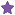• Posts: 5004
• Country:• Reputation: 85##### Re: error
« Reply #1 on: July 16, 2021, 21:51 »
Looks to me like you are trying to run a counterpoise-corrected calculation, but you haven't actually specified which atoms belong to the two parts of the system. But it's hard to tell exactly without seeing the input file.

Also I find it odd that these error messages appear more than once, but maybe there is a good reason for it. Just make sure that when you run in parallel, you use the mpiexec that we ship with the software.

####A_Shimizu

• New ATK user
•• Posts: 4
• Country:• Reputation: 0##### Re: error
« Reply #2 on: July 25, 2021, 21:28 »